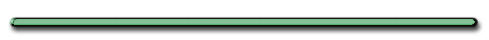HomeTry This at Home[ HTML5 ]
(d vs t)Video Instruction

showmethephysics.com

B. Interpreting Motion Diagrams

Acceleration Review

Acceleration - the time rate of change in velocity

a = ∆V/∆t = (Vf - Vi)/t

 Uniform Acceleration?

- constant  change
in velocity per unit time

Which data shows uniform acceleration? Why?

 time (sec) Velocity (m/s) 0 5 1 7 2 9 3 11 4 13
 time (sec) Velocity (m/s) 0 5 1 10 2 14 3 20 4 30

Left Data Shows Constant Acceleration

a = ?

a = 2 m/s2

Non-Constant OR Constant Acceleratio?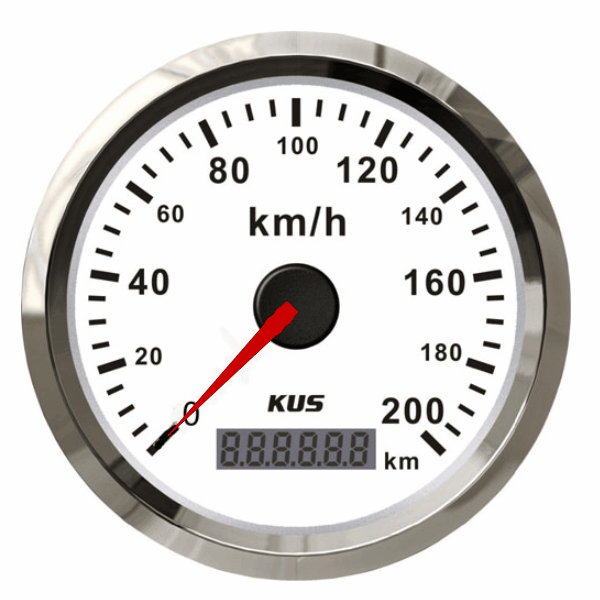Ex) Describe the accelerationacceleration is constant +

Constant Non-Constant Acceleration?Prove this is constant accelerationEvery 2 seconds the object gains exactly 15 m/s

Motion Map

Velocity

0 m/s / 15 m/s / 30 m/s / 45 m/s0 sec / 2 sec / 2 sec / 2 secFind the acceleration

a = ∆V/∆t

(+15 m/s)/2 sec

a = +7.5 m/s2Motion Map

Acceleration

2 sec / 2 sec / 2 sec / 2 sec+7.5 m/s2/+7.5 m/s2/+7.5 m/s2/+7.5 m/s2

Motion Map

DisplacementSlope of a V vs. t plot

Slope = ∆y/∆x

= ∆V/∆t = a

Ex)

Describe motion using
y axis and slope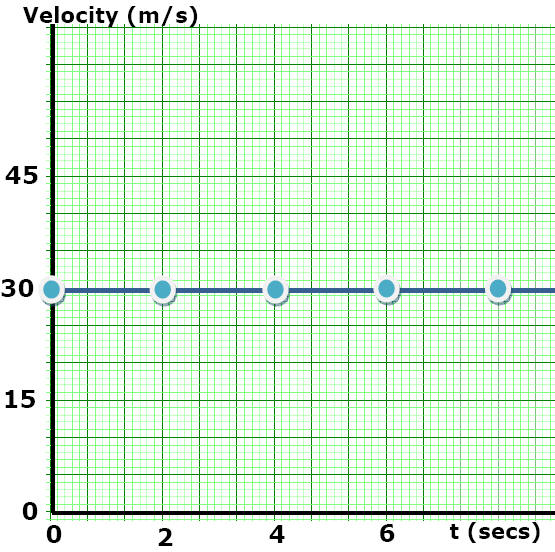Step 1: y axis:

Step 2: Slope

Step 1: y axis: V constant

Step 2: Slope = ∆V/∆t  = a

a = 0Ranking Task Rank the acceleration from largest to smallestJanuary 2001 Regents

 Ex 4)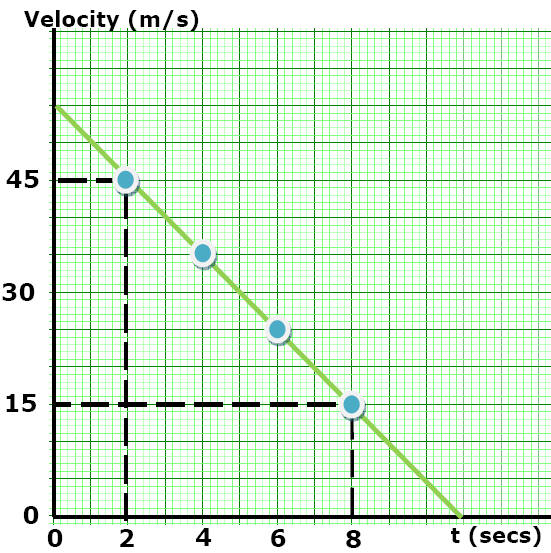Step 1: y axis:     Step 2: Slope   Answers   Velocity is Decreasing,   Acceleration is constant, negative     Calculate the accelerationa = (Vf- Vi)/t      (15 m/s - 45 m/s )     (8 sec - 2 sec)      = -5 m/s2     Video Instruction showmethephysics.com        Ex 5) Describe the motions Velocity (m/s)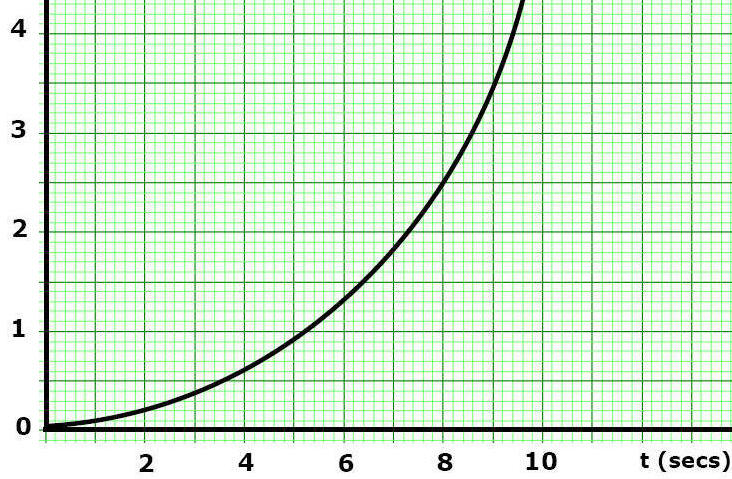Step 1: y axis: Step 2: Slope   Answers Step 1: y axis:  V increasing     Step 2: Slope = ∆V/∆t  = a a is increasing   Ex) Describe the motions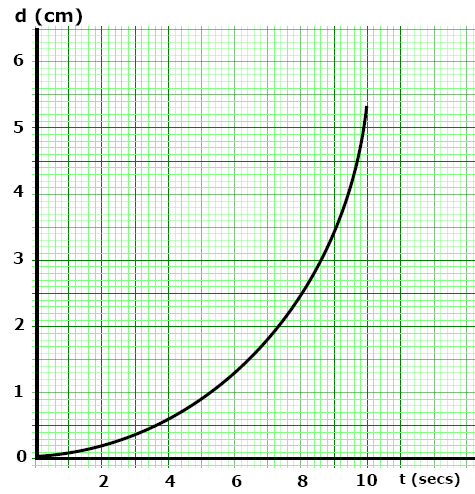Step 1: y axis: Step 2: Slope           Step 1: y axis: d increasing Step 2: Slope = d/t = V   Velocity is increasing smoothly  Acceleration is constant & Positive   Non-Uniform AccelerationEx) Describe motionsStep 1: y axis: Step 2: Slope           Step 1: y axis: d increasing Step 2: Slope = d/t = v Velocity is Decreasing smoothly (slope)   Acceleration is constant & negativeDescribe motions d (cm)Step 1: y axis: Step 2: Slope      Step 1: y axis: d constant Step 2: Slope = d/t = v   v = 0 (slope) a = 0         Analyzing Motion Plots - HTML5 Show Me The PhysicsSummary©Tony Mangiacapre., - All Rights Reserved [Home] Established 1995 Use any material on this site (w/ attribution)